nextnano.com  GUI: nextnanomat  Tool: nextnano++  Tool: nextnano³  Tool: nextnano.NEGF  Download | Search | Copyright | News | Publications  * password protected nextnano³ software1D RTD (NEGF)

# nextnano3 - Tutorial

## Ballistic current in a resonant tunneling diode (RTD)

Author: Stefan Birner, Tillmann Kubis

If you are interested in the input files that are used within this tutorial, please contact stefan.birner@nextnano.de.
```1) -> 1D_RTD_AlGaAs_GaAs_green.in  ```

`==> `Please see also this RTD tutorial.

## Ballistic current in a resonant tunneling diode (RTD)

This input file for the Resonant Tunneling Diode (RTD) is based on the paper of

Self-consistent quantum transport theory: Applications and assessment of approximate models
T. Kubis, P. Vogl
Journal of Computational Electronics 6, 183 (2007)

The resonant tunneling structure has the following properties:

• device length: 40 nm
• two 3 nm Al0.3Ga0.7As barriers separated by a 4 nm GaAs quantum well in the center
• 16 nm intrinsic region:
`- `3 nm left and right of the barriers, 3 nm + 3 nm in barriers and 4 nm in well
• 12 nm n-doped region left and right of the intrinsic region with n = 2 * 1017 cm-3
• conduction band offset: CBO(GaAs/Al0.3Ga0.7As) = 230 meV
• electron masses:
`- `GaAs:           me = 0.067 m0
`- `Al0.3Ga0.7As: me = 0.0919 m0
• temperature: 300 K
• grid resolution: 1 nm (homogeneous grid)

The method of calculating the carrier transport is defined as "fully self-consistent nonequilibrium Green's function (NEGF) approach for vertical quantum transport in open quantum devices with contacts". For the purpose of this tutorial (to make the calculations orders of magnitudes faster), we do not include scattering, thus the method of calculating the current is termed "ballistic transport".

The electrons are described within a one-band model with a variable effective mass, i.e. a spatially dependent (= material dependent) effective electron mass me(z).

The following figure shows the IV characteristics of the resonant tunneling diode.
The resonance peak (resonant tunneling) is at an applied voltage of 170 mV.
This current-voltage (I-V) characteristic shows a negative differential conductivity which can be used in amplifiers or oscillators.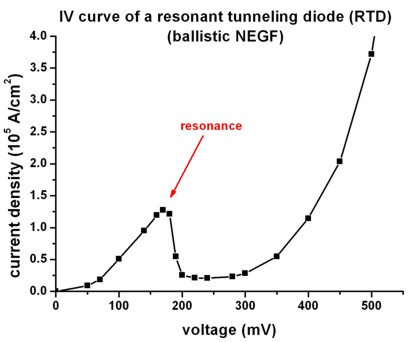The applied bias modifies the Fermi levels, shifts the energy of the resonant state and changes the conduction band profile due to charge redistributions (Poisson equation), and thus alters the transmission properties of the barriers.

An important quantity of RTDs is the "peak-to-valley" ratio of the current which is defined as the current density in resonance jr divided by the current density in the valley jv. For our ballistic simulation we obtain a peak-to-valley ratio of jp/jv  = 6.

Schrödinger equation with Dirichlet boundary conditions at 0 nm and 40 nm

The following figure shows the self-consistently calculated conduction band profile of the GaAs/AlGaAs resonant tunneling structure plotted at the resonant bias voltage of 170 mV including the wave functions (psi2). Note that these wave functions do not necessarily correspond to reality as we imposed artificially high (i.e. infinite) barriers to the left and right side of the sample region by applying Dirichlet boundary conditions to the Schrödinger equation (i.e. psi = 0 at 0 nm and at 40 nm). We also note that these wave functions are not used in the NEGF formalism. They are only shown for a qualitative understanding of the structure.
Nevertheless, one can see the two states that are interesting in understanding the device a bit more clearly.
In front of the left barrier of any RTD under forward bias, a triangular quasi-bound is formed.
In the ballistic case, the triangular quasi-bound state is below the left contact and is thus irrelevant for the conductance whereas the resonance state is responsible for the peak in the I-V characteristic. If one increases the voltage further, the resonance state will move below the energy of the left contact and will then become irrelevant for the conductance, similar to the triangular quasi-bound state. In that case, the current drops into the valley of the I-V characteristic.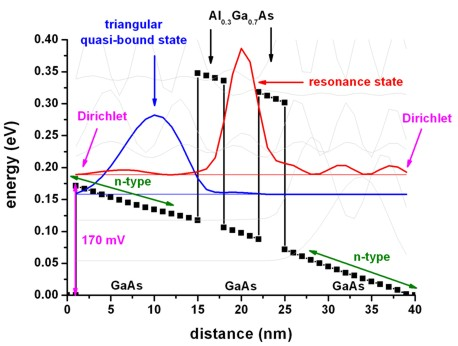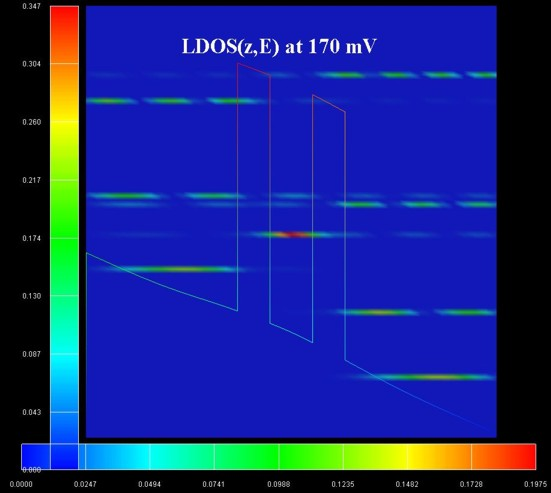The right figure shows the local density of states LDOS(z,E) of the simulated structure. Note that the Schrödinger equation for this structure was simulated with Dirichlet boundary conditions (psi = 0) at z = 0 nm and z = 40 nm. This will lead to artificially confined states that are not present in a simulation where "open boundaries" are used.
(Note: The LDOS figure does not include the factor of 2 due to spin degeneracy. The code has been modified meanwhile. The factor of 2 is now included in the LDOS outout.)

Schrödinger equation with Dirichlet boundary conditions at -300 nm and 340 nm

A more realistic picture of the wave functions can be obtained by calculating the wave functions for the same structure but this time including a flat-band region of 300 nm region to the left and to the right of the structure mimicking some kind of "open boundaries".
One can still see the triangular quasi-bound state which looks nearly identical to the structure with the Dirichlet boundary conditions at 0 nm and 40 nm.
The resonance states, however, look differently. The resonance states couple to the states in the contacts more strongly and now there are several of them. This corresponds to a broadening of the resonance energy.

This explains why a model that takes into account "open boundary conditions" (like our NEGF formalism) is very important in modeling transport.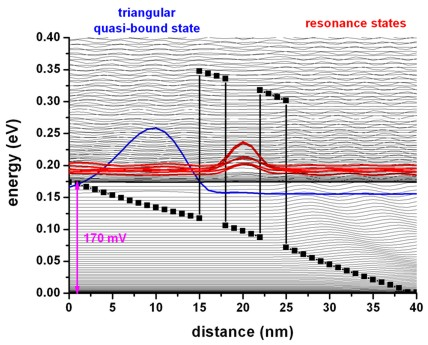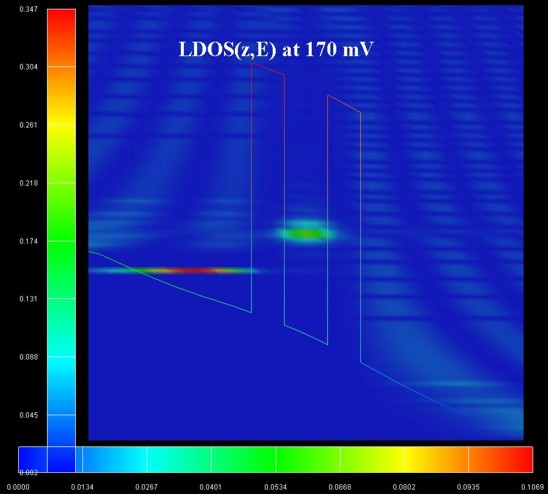The right figure shows the local density of states LDOS(z,E) of the simulated structure. Note that the Schrödinger equation for this structure was simulated with Dirichlet boundary conditions (psi = 0) at z = `-`300 nm and z = 340 nm. This large quantum cluster mimicks open boundary conditions, i.e. the states in the central RTD region are not influenced significantly by the boundary conditions. The units of the LDOS are ` [nm-1 eV-1]`.
(Note: The LDOS figure does not include the factor of 2 due to spin degeneracy. They code has been modified meanwhile. The factor of 2 is now included in the LDOS outout.)

## Energy resolved electron density and energy resolved local density of states (LDOS)

The following figure shows the self-consistently calculated conduction band profile of the GaAs/AlGaAs resonant tunneling structure plotted at the resonant bias voltage of 170 mV together with
`- `(left   figure) the energy resolved electron density n(z,E) as a contour plot in units of` [eV-1 Angstrom``-3````] = [eV-1 1024 cm-3]```.
`  ==> EnergyResolvedDensity_avs.fld   , *.coord, *.dat`
`  `Note that the horizontal legend is relevant for the color map of the energy resolved electron density.
`- `(right figure) the spectral function (energy resolved local density of states LDOS(z,E)) is shown as a contour plot in units of` [eV-1 Angstrom``-1``]`.
`  ==> spectral_real_avs.fld, *.coord, *.dat`
`  `Note that the horizontal legend is relevant for the color map of the energy resolved local density of states.

The vertical legend refers to the color map of the conduction band profile. The maximum value of the conduction band edge can be read directly from this legend. The right most point is at 0 eV.

The right figure shows that the triangular quasi-bound state has a high local density of states. As expected and which can be seen in the left figure, this state is not occupied with electrons. It can be nicely seen that the states from the left contact are in resonance with the quantum well resonance states.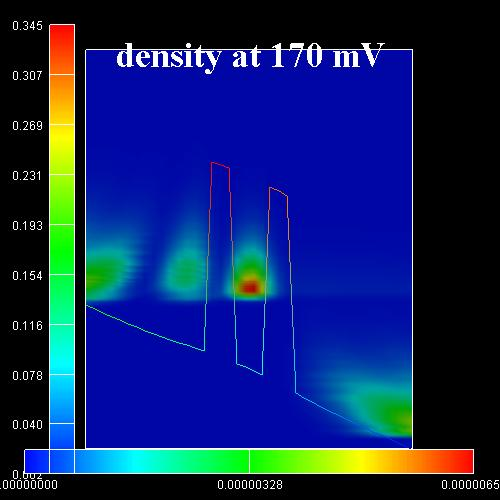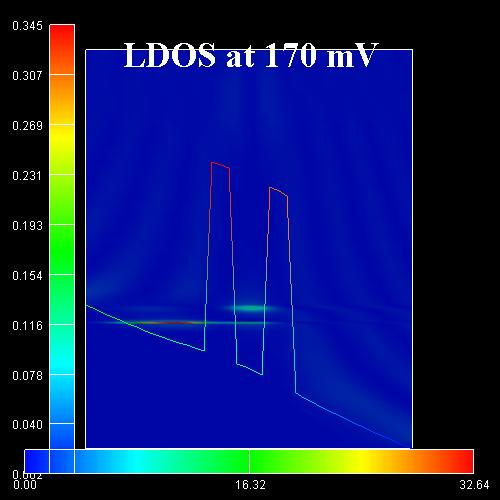Further below are the same plots at different biases: 70 mV, 200 mV (voltage of valley of I-V characteristics), 400 mV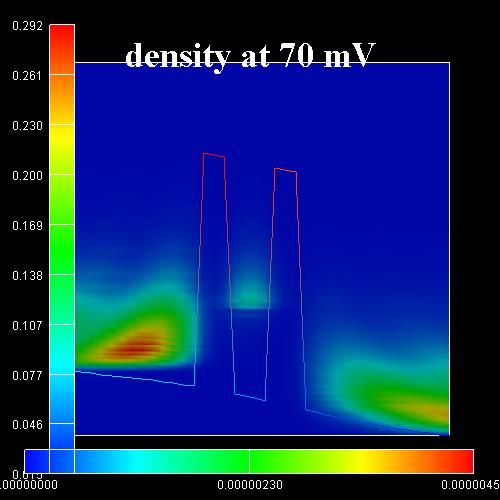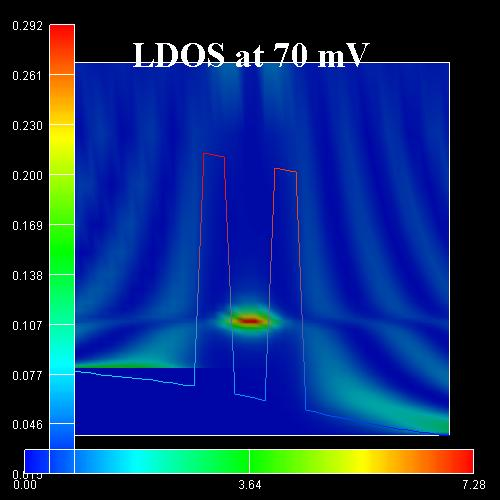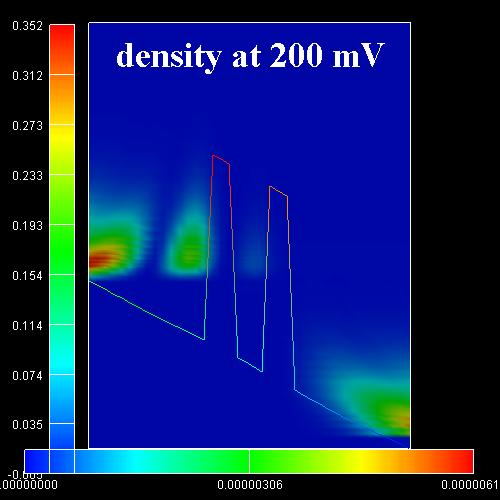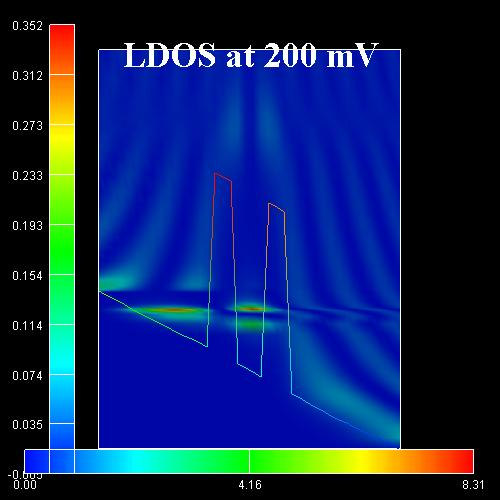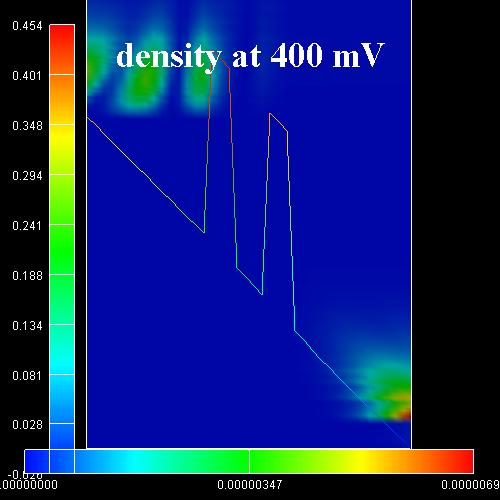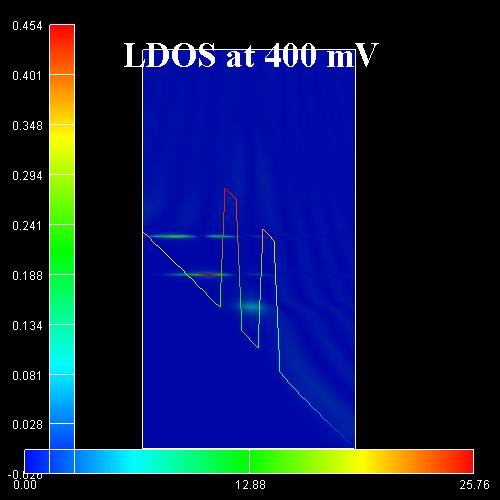### Including scattering

T. Kubis and P. Vogl showed in their above cited paper, that due to scattering, the triangular quasi-bound state will be filled by electrons that have a "high" energy at the left contact, and then lose energy by scattering events and thus fill this triangular bound-state. The peak current in the I-V characteristic then occurs at a bias voltage where this quasi-bound state is aligned with the resonant quantum well state.

Assuming ballistic transport, the electrons in the contact fly towards the barrier, maintaining their (high) energy and therefore hit the resonance energy in the well at a significantly lower bias as compared to a calculation including scattering where the electrons lose energy to occupy the triangular quasi-bound state. In the ballistic case, the I-V characteristic is then entirely determined by the properties of the contact rather than by the doping of the device. A careful inclusion of all inelastic processes is therefore necessary to capture the physical mechanisms that determine the resonance bias and peak (and valley) value of the RTD.

For the results of the calculations including scattering (and a discussion of it), we refer to the section 4 of the above mentioned paper:

Self-consistent quantum transport theory: Applications and assessment of approximate models
T. Kubis, P. Vogl
Journal of Computational Electronics 6, 183 (2007)

• Please help us to improve our tutorial. Send comments to``` support [at] nextnano.com```.